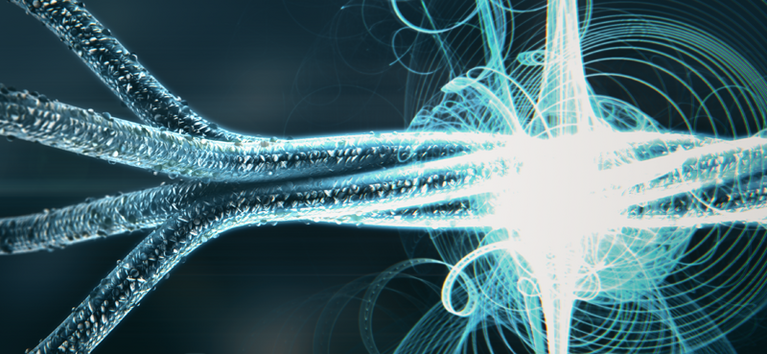## P17 – Quantum many-body systems and tensor networks for quantum computation

### Abstract:

The goal of this project is to develop a toolbox for utilizing quantum many-body problems as use cases for near-term quantum computers and simulators. We will develop improved schemes to prepare quantum many-body states on quantum computers and simulators, as well as to verify the prepared states, taking into account the given levels of control and precision. We will further devise use cases for those preparation schemes, both in simulating quantum problems, and by encoding classical tasks into quantum problems (such as tensor contractions, relevant e.g. in machine learning). We will complement this by advancing classical simulation of quantum computing through the design of hybrid simulation methods which combine tensor networks with other simulatable classes, and which we will apply towards the simulation of the aforementioned problems. A key novelty lies in the unique combination of methods from quantum information, quantum many-body physics, and mathematical physics which we will employ to tackle the problem in a comprehensive fashion. The outcomes of the project will contribute towards the goals of the SFB BeyondC by supplying us with a wide range of tools for the preparation, verification, simulation, and application of quantum many-body problems in near-term quantum computers and quantum simulators.

PI Norbert Schuch on
Quantum many-body systems and tensor networks for quantum computation